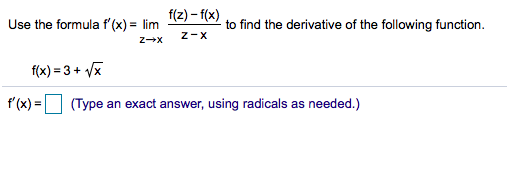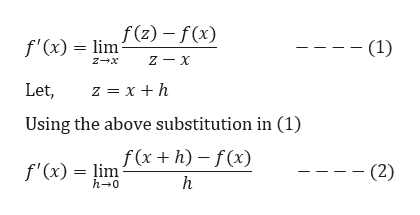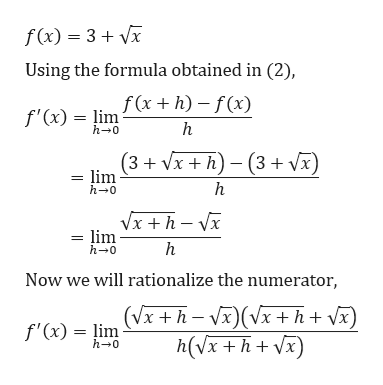# Use the formula f'(x)= lim (2)-f(x) to find the derivative of the following function.Z-Xf(x) 3+ x(Type an exact answer, using radicals as needed.)f(x)

Question
8 viewshelp_outlineImage TranscriptioncloseUse the formula f'(x)= lim (2)-f(x) to find the derivative of the following function. Z-X f(x) 3+ x (Type an exact answer, using radicals as needed.) f(x) fullscreen
check_circle

Step 1

Given,help_outlineImage Transcriptionclosef'(x)= lim2) - f(x) - (1) Let, z xh Using the above substitution in (1) f'(x) limx+h)-f(x) h h 0 - (2) fullscreen
Step 2

Given,

...help_outlineImage Transcriptionclosef(x) 3Vx Using the formula obtained in (2), f'(x) = lim (x+h)- f(x) h h-0 (3+x+h) (3+ vx) lim h 0 h Vx + h - vx lim h-0 h Now we will rationalize the numerator, (Vx+h x+h+vx) h(VxhVx) f'(x) lim h 0 | fullscreen

### Want to see the full answer?

See Solution

#### Want to see this answer and more?

Solutions are written by subject experts who are available 24/7. Questions are typically answered within 1 hour.*

See Solution
*Response times may vary by subject and question.
Tagged in

### Calculus